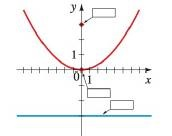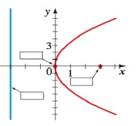Chapter 12.1, Problem 4E### Algebra and Trigonometry (MindTap ...

4th Edition
James Stewart + 2 others
ISBN: 9781305071742

#### Solutions

Chapter
Section### Algebra and Trigonometry (MindTap ...

4th Edition
James Stewart + 2 others
ISBN: 9781305071742
Textbook Problem

# Label the focus, directrix, and vertex on the graphs given for the parabolas in Exercises 2 and 3. (a) x 2 = 12 y(b) y 2 = 12 xTo determine

(a)

To label:

The focus, directrix and vertex on the graph.

Explanation

Given:

The graph of the parabola x2=12y is given as,

figure (1)

Approach:

The equation of a parabola is given by,

x2=4py … (1)

And, the properties for the parabola are as follows,

Vertex =V(0,0).

Focus =F(0,p).

Directrix y=p.

Calculation:

Consider the given equation.

x2=12y

Compare the above equation with equation (1).

To determine

(b)

To label:

The focus, directrix and vertex on the graph.

### Still sussing out bartleby?

Check out a sample textbook solution.

See a sample solution

#### The Solution to Your Study Problems

Bartleby provides explanations to thousands of textbook problems written by our experts, many with advanced degrees!

Get Started